# NTSE Level Test: The Human Eye & the Colourful World

## 25 Questions MCQ Test NCERT Textbooks (Class 6 to Class 12) | NTSE Level Test: The Human Eye & the Colourful World

Description
Attempt NTSE Level Test: The Human Eye & the Colourful World | 25 questions in 25 minutes | Mock test for Class 10 preparation | Free important questions MCQ to study NCERT Textbooks (Class 6 to Class 12) for Class 10 Exam | Download free PDF with solutions
QUESTION: 1

### Which is the correct condition for the total internal reflection to occur ?

Solution:

The light must be travelling from a more dense medium into a less dense medium the angle of incidence must be greater than the critical angle.

QUESTION: 2

### If the angle of incidence is increased for a pair of air – glass interface, then the angle of refraction will

Solution:

As the angle of incidence increases, the angle of refraction also increases proportionally to the increase of incidence.
( By Snell's law) .

QUESTION: 3

### The lateral displacement of an incident ray passing out of a rectangular glass slab

Solution:

The lateral displacement of an incident ray passing out of a rectangular glass slab  is directly proportional to the thickness of glass slab, angle of incidence, and refractive index however it is inversely proportional to the wavelength of incident light.

QUESTION: 4

Find the incorrect statement

Solution:
QUESTION: 5

A person developing near sightedness due to-

Solution:
QUESTION: 6

Which of the following phenomena is not the result of total internal reflection ?

Solution:

Twinkling of stars is due to atmospheric refraction. Distant star acts like a point source of light. When the Starlight enters the earth's atmosphere it undergoes refraction continuously, due to changing refractive index ie. From rarer to denser medium.

QUESTION: 7

Danger signals are red in colour because

Solution:
QUESTION: 8

Optical fibre is used for

Solution:
QUESTION: 9

A person cannot see distinctly any object placed beyond 40cm from his eyes. Calculate the power of the lens which will help him to see distant objects clearly.

Solution:

A lens which can bring an object at infinity to 40 cm is required here.
So, u = infinity, v = -40 cm
We know, 1/f  = 1/ v - 1/u
1/ f  = 1/-40 - 1/infinity
So, f = -40 cm = -0. 4 m
Power of lens, P = 1/ f = 1/ -0. 4 = -2. 5 D

QUESTION: 10

Bees are sensitive to

Solution:
QUESTION: 11

Statement A : Improper functioning of rod shaped cells causes colour blindness
Statement B : The focal length of the human eye can be increased or decreased

Solution:
QUESTION: 12

A boy uses spectacles of focal length – 50cm. Hence the defect of vision, he is suffering from-

Solution:
QUESTION: 13

What happens to the image distance in the eye when we increase the distance of an object from the eye ?

Solution:
QUESTION: 14

The near point of a hypermetropic person is 75 cm if the person uses eye glasses having power +1.0D, Calculate the distance of distinct vision for him ?

Solution:

u = -75 cm
P = 1/f
f = 1 m = 100 cm
So, by lens formula ;
1/f = 1/v - 1/ u
So, 1/ v = 1/f +1/u
1/ v =  1/100 + (-1/75)
1/v = -1/300
v = -300 cm

QUESTION: 15

What is the value of refractive index of the medium if the critical angle of incidence in a denser – rarer inter face is equal to 45° ?

Solution:

Sine(Critical angle) = 1/refractive index so sin 45 = 1/1.41/1.4 = 1/ refractive index hence refractive index = 1.44

QUESTION: 16

Match the following with correct response.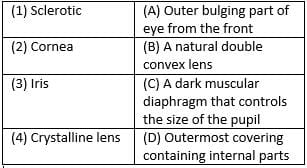Solution:
QUESTION: 17

Match the following with correct response.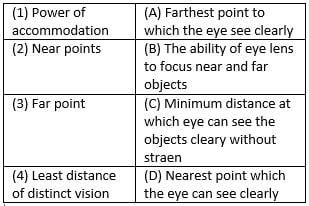Solution:

The ability of eye lens to focus near and far objects is called power of accommodation.
Nearest point which the eye can see clearly is called near points.
The farthest point to which the eye see clearly is known as far point.
Least distance of distinct vision is the minimum distance at which eye can see the object clearly without strain.

QUESTION: 18

Match the following with correct response.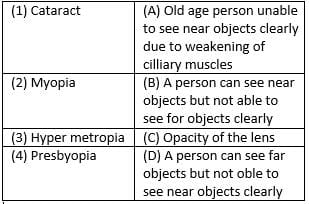Solution:
QUESTION: 19

Match the following with correct response.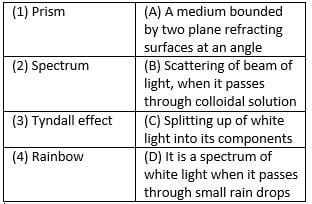Solution:

A medium bounded by two plane refracting surfaces at an angle is known as prism.
Splitting up of white light into its components is called spectrum.
Scattering of beam of light, when it passes  through colloidal solution is called Tyndall effect. Rainbow is a spectrum of white light when it passes through small rain drops.

QUESTION: 20

Match the following with correct response.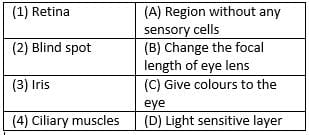Solution:
QUESTION: 21

Match the following with correct response.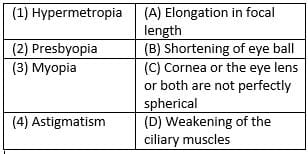Solution:
QUESTION: 22

Match the following with correct response.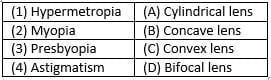Solution:
QUESTION: 23

Match the following with correct response.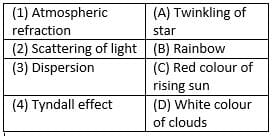Solution:
QUESTION: 24

Match the following with correct response.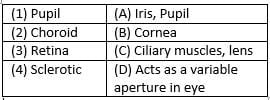Solution:
QUESTION: 25

Which of following phenomena based on atmospheric refraction and total internal reflection
A. Sun appears to rise 2 minutes before and 2 minutes later
B. Stars seen higher than they actually are
C. Rainbow
D. Blue colour of clear sky

Solution:

Advanced sunrise and delayed sunset are phenomena caused due to atmospheric refraction. In this the sun appears to rise early by 2 minutes and set late by 2 minutes.
A rainbow is a meteorological phenomena that is caused by total internal reflection refraction and dispersion of light in water droplets resulting in a spectrum of light appearing in the sky.Use Code STAYHOME200 and get INR 200 additional OFF Use Coupon Code